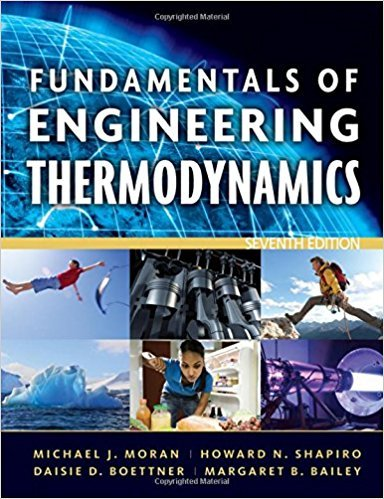×
×

# Solution: Reviewing ConceptsAnswer the following true orISBN: 9780470495902 50

## Solution for problem 97P Chapter 2

Fundamentals of Engineering Thermodynamics | 7th Edition

• Textbook Solutions
• 2901 Step-by-step solutions solved by professors and subject experts
• Get 24/7 help from StudySoup virtual teaching assistantsFundamentals of Engineering Thermodynamics | 7th Edition

4 5 1 405 Reviews
30
4
Problem 97P

Reviewing Concepts

Answer the following true or false. Explain.

(a) Work is done by a system on its surroundings if the sole effect on everything external to the system could have been the raising of a weight.

(b) Cooling of computer components achieved by a fan-induced flow of air falls in the realm of radiation heat transfer.

(c) For every thermodynamic cycle, the net amounts of energy transfer by heat and work per cycle are equal.

(d) A flywheel spinning owing to an input of electricity stores energy as internal energy.

(e) Kinetic and potential energy are each extensive properties of a system.

Step-by-Step Solution:

Step 1 of 6</p>

We have to identify true/ false of the sentences.

Step 2 of 6</p>

a)It is true because the work done is the amount of force required to change the position of the object..

The raising of weight=Force acting through a distance

Step 3 of 6</p>

b)False. it is not a radiation transfer.It is convection heat transfer because medium is present in this process of heat transfer.

Step 4 of 6

Step 5 of 6

##### ISBN: 9780470495902

The full step-by-step solution to problem: 97P from chapter: 2 was answered by , our top Engineering and Tech solution expert on 07/20/17, 09:01AM. Fundamentals of Engineering Thermodynamics was written by and is associated to the ISBN: 9780470495902. The answer to “Reviewing ConceptsAnswer the following true or false. Explain.(a) Work is done by a system on its surroundings if the sole effect on everything external to the system could have been the raising of a weight.________________(b) Cooling of computer components achieved by a fan-induced flow of air falls in the realm of radiation heat transfer.________________(c) For every thermodynamic cycle, the net amounts of energy transfer by heat and work per cycle are equal.________________(d) A flywheel spinning owing to an input of electricity stores energy as internal energy.________________(e) Kinetic and potential energy are each extensive properties of a system.” is broken down into a number of easy to follow steps, and 97 words. This full solution covers the following key subjects: Energy, system, heat, work, transfer. This expansive textbook survival guide covers 14 chapters, and 1501 solutions. Since the solution to 97P from 2 chapter was answered, more than 240 students have viewed the full step-by-step answer. This textbook survival guide was created for the textbook: Fundamentals of Engineering Thermodynamics, edition: 7.

Unlock Textbook Solution